## 3.10.2 Master Equation for the Density Matrix

The evolution for the density matrix is described by a quantum LIOUVILLE equation.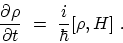(3.91)

To simplify the equation, the limit of weak interaction between the device and the heat bath is applied. Coarse-graining in time results in a MARKOVian system. The resulting quantum LIOUVILLE equation for the reduced density matrix is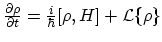and describes the quantum evolution of a system with loss .

Irreversible or energy-dissipating processes always involve transitions between quantum states. Such processes are described, at the simplest level, by a master or rate equation. The time evolution of such systems is determined by the rates of transition between states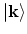. These rates are usually estimated using the FERMI golden rule(3.92)

where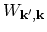is the transition rate from the state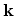to the state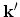. The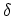function ensures energy conservation. If one assumes that these transitions occur independently within any small time interval (the MARKOV assumption), the transition rate from stateto statewill produce changes in the corresponding occupation factors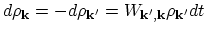. The occupation of the stateincreases and that of the statedecreases as a result of this particular process, and the amount of change depends only on the occupation of the initial state3.1. If one sums over all possible transition processes, one obtains the master equation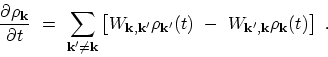(3.93)

The PAULI master equation  is a frequently used model of irreversible processes in simple quantum systems. It can be derived from elementary quantum mechanics along with a MARKOV assumption.

In order to evaluate transport, the device under consideration must be coupled to external reservoirs. Coupling introduces carrier exchange between device and reservoirs, which are assumed to be in thermal equilibrium. The difference between the electrochemical potentials of the reservoirs causes current through the device. Therefore, it is essential to properly include the coupling to the reservoirs in the master equation.

In the the PAULI master equation approach developed by FISCHETTI, this coupling is introduced in a phenomenological manner [213,214]. Application of the PAULI master equation is restricted to stationary systems, since in the non-stationary case the current continuity would be violated . Another issue is that the PAULI master equation can only be justified for devices where the quantum region is shorter than the phase coherence length .

A solution free from the above mentioned shortcoming of phenomenological coupling of the device to the reservoirs was suggested by GEBRAUER and CAR [216,217]. They impose periodic boundary conditions upon the unperturbed system. This approach can be also used to describe transients.

M. Pourfath: Numerical Study of Quantum Transport in Carbon Nanotube-Based Transistors